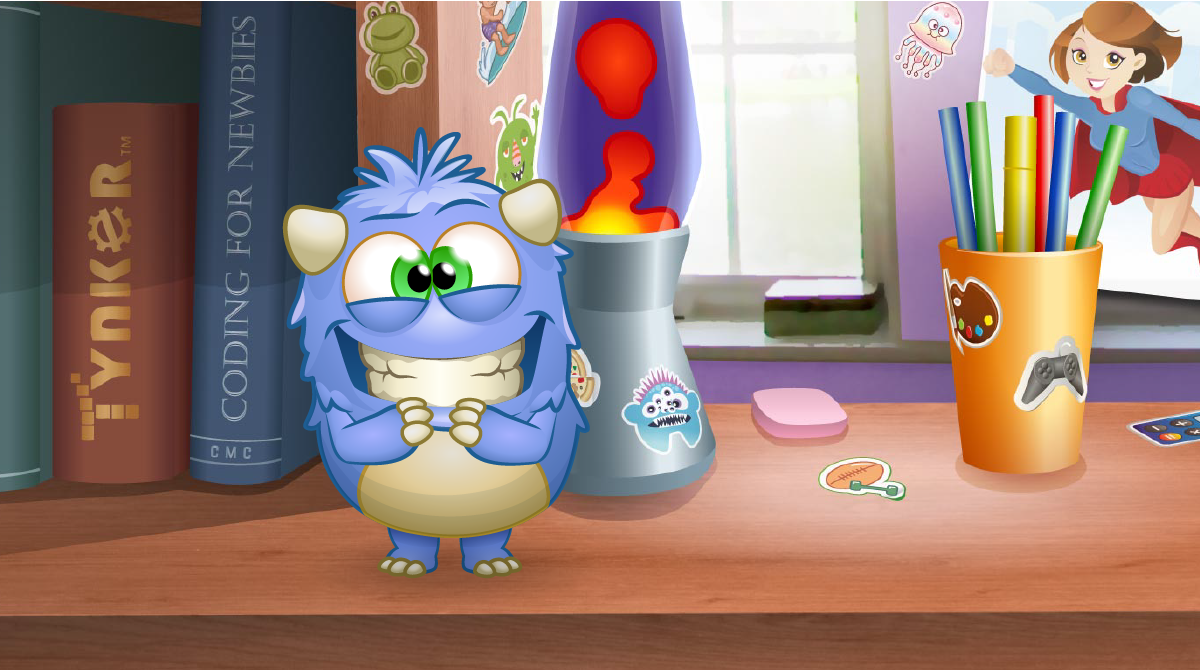# Programming 1A Icon Coding 7-Course Pack Space Cadet Dragon Spells Programming 100 Programming 300 Barbie™ You Can Be Anything™ Programming 1A Programming 1B Programming 101 Programming 102 Programming 201 Programming 202 Programming 301 Programming 302 JavaScript 101 Python 101 Web Development 101 Python 201 Drones 101 WeDo Coding Augmented Reality micro:bit 101 MicroPython 101 Life Science Physical Science Earth Science Math Social Studies English Life Science Physical Science Earth Science Math Social Studies English

This course is part of Coding/STEAM Curriculum - K-8 Plan

K-8 Plan
\$3,800 per year

### Programming 1AA puzzle-based introduction to coding concepts for beginners where they learn sequencing, pattern recognition, loops, and conditional logic. Request Quote

##### Course Summary
• Beginner level
• 12 lessons
• Tynker Blocks
• Web
##### Course Includes
• 12 lessons
• 62 activities
• Enhanced Creativity Tools
• Automatic Assessment
• Tutorials and Reviews
• Coding Puzzles
##### Prerequisites
No previous coding experience required.

## Lesson Plan

### Introduction

Students are introduced to rotation measured in degrees and moving forward in units as they program a racer to follow the green lines and collect orbs.

### New Code Blocks

• : Move the Actor a specified number of units forward. Note this "forward" block is set to the value "400," so the code will move the Actor 400 units forward.

• : Rotate the Actor to the right (clockwise) a specified degree of rotation. Note this "turnRight" block is set to the value "90," so the code will rotate the Actor 90 degrees right.

• : Rotate the Actor to the left (counter-clockwise) a specified degree of rotation. Note this "turnLeft" block is set to the value "90," so the code will rotate the Actor 90 degrees left.

### Vocabulary

• Angle: The amount of turn between two straight lines that meet together at the same point
• Degrees: A unit of measurement used to describe the size of angles
• Right Angle: Angle which is equal to 90 degrees
• Square: A four-sided shape where all sides have the same length, and all angles are right angles (90 degrees)
• Rectangle: A four-sided shape where all where all opposite sides have the same length, and all angles are right angles (90 degrees)

### Objectives

Students will...
• Use rotation to navigate a racer
• Apply commands in a sequence to draw shapes

### Warm-Up(20 minutes)

1. Say, “Today we’re going to learn degrees of rotation.”
2. Draw a circle on the classroom board and divide it into four equal slices. Write “0 degrees” at the top and “90 degrees” at the right:3. Say, “From the top of the circle to right of the circle is 90 degrees.” Explain that an angle of 90 degrees is called a “right angle.”
4. Ask, "How many degrees do I need to turn to see what’s to my right?" (90 degrees right)
5. Ask, "How many degrees do I need to turn to see what’s to my left? (90 degrees left)
6. Draw and label the following shapes on the classroom board:7. As a class, label each 90 degree angle in the square and rectangle.
8. Ask, “How many degrees does a right angle have?” (90 degrees)

### Getting Started(20 minutes)

As a class, solve “Module 3: Right Angle.”
1. Open "Module 3: Right Angle."
2. Explain that the goal of these puzzles will be to help the racer trace the green lines.
3. Remind students to think of the “turn right” code block as “rotate right.”
4. Tell students to focus on the grid lines. Say, “The grid lines start at zero and increase by 100.”
5. Explain that “forward 400” moves the racer 400 units forward in whatever direction it’s facing.
6. As a class, solve the puzzle: forward 400 - turn right 90 degrees - forward 400

### Activities(35 minutes)

##### 1. Right Angle (Puzzle)
• Explain that the goal is to trace the “L” shape.
• Remind students that “forward” 400 moves the racer 400 units forward in whatever direction it’s facing.
• Remind students that “turnRight 90” rotates the racer 90 degrees right in whatever direction it’s facing.
##### 2. Another Right Angle (Puzzle)
• Remind students that “turnLeft 90” rotates the racer 90 degrees left in whatever direction it’s facing.
##### 3. Square (Puzzle)
• Tell students they will have to use several “forward” and “turnRight” blocks to trace a square with the racer. Remind students of the warm-up and how many 90 degree angles are in a square.
• Students who get stuck may not understand that the racer needs to move forward before it can turn. Help them understand this concept by acting out the steps before using any code blocks.
##### 4. Rectangle (Puzzle)
• The “forward” code block has a value of 400, so students will have to change the value to trace the two shorter sides of the rectangle.
• Tell students to click the number inside the “forward” block to change it from 400 to 200.

### Optional Activities(15 minutes)

Review
• How many degrees make a right angle?
• How many right angles does a square have? A rectangle? (four 90 degree angles)
• What is one piece of advice you would give someone using the “turnRight 90” code block?

### Standards

CCSS-ELA: RI.1.1, RI.1.6, RI.1.10, RF.1.4, RF.1.4.A, RF.1.1, SL.1.1, SL.1.2, SL.1.3, RI.2.1, RF.2.4, RF.2.4.A, RI.2.6, SL.2.1, SL.2.2, SL.2.3
CCSS-Math: MP.1, 1.OA.B.3, 1.GA.1, 2.G.A.1, 2.OA.B.2, 2.NBT.A.1.A, 2.GA.1, 2.GA.2
CSTA: 1A-AP-09, 1A-AP-11, 1A-AP-15
CS CA: K-2.AP.13, K-2.AP.14, K-2.AP.17
ISTE: 1.c, 1.d, 4.d, 5.c, 5.d, 7.c